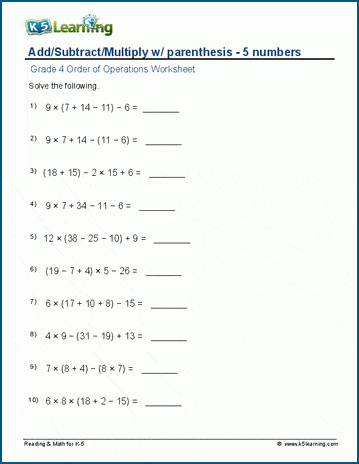# Add/subtract/multiply with parenthesis - 5 numbers

## Order of operations worksheets: Adding, subtracting and multiplying with parenthesis (5 numbers)

Below are six versions of our grade 4 math worksheet on order of operations.  Equations combine adding, subtracting and multiplying numbers with parenthesis. Each question has 5 positive whole numbers. These worksheets are pdf files.## More order of operations worksheets

Browse all of our order of operations worksheets, from adding and subtracting with parenthesis to full PEMDAS worksheets including exponents.

## What is K5?

K5 Learning offers reading and math worksheets, workbooks and an online reading and math program for kids in kindergarten to grade 5.  We help your children build good study habits and excel in school.